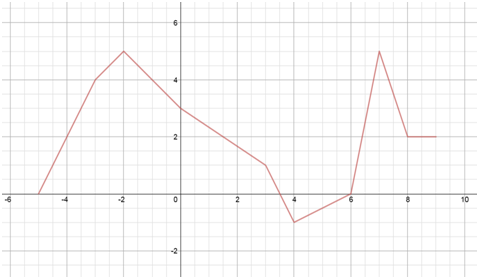# Function notation (advanced)

### Function notation (advanced)

#### Lessons

• 1.
Introduction to Function Notation
If $f(x) = 5x^2-x+6$ find the following
a)
${f(\heartsuit)}$

b)
${f(\theta)}$

c)
${f(3)}$

d)
${f(-1)}$

e)
${f(3x)}$

f)
${f(-x)}$

g)
${f(3x-4)}$

h)
${3f(x)}$

i)
${f(x)-3}$

• 2.
Express a Function as $f($
$)$

If ${f(x) = \sqrt{x},}$ write the following in terms of the function ${f.}$
a)
${\sqrt{x}+5}$

b)
${\sqrt{x+5}}$

c)
${\sqrt{2x-3}}$

d)
${-8\sqrt{x}}$

e)
${-8\sqrt{2x-3}}$

f)
$4\sqrt{x^{5}+9}-1$

• 3.
Find the Value of a Function from Its Graph
Find the value of the following from the given grapha)
${f(3)}$

b)
${f(0)}$

c)
${f(-5)}$

d)
${f(x)=5,x=?}$

e)
${f(x)=-1,x=?}$

f)
${f(x)=0,x=?}$